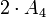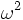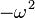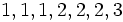Linear representation theory of special linear group:SL(2,3)

This article gives specific information, namely, linear representation theory, about a particular group, namely: special linear group:SL(2,3).
View linear representation theory of particular groups | View other specific information about special linear group:SL(2,3)

Summary

Item Value
degrees of irreducible representations over a splitting field (such as$\mathbb{C}$ or$\overline{\mathbb{Q}}$) 1,1,1,2,2,2,3
grouped: 1 (3 times), 2 (3 times), 3 (1 time)
maximum: 3, lcm: 6, number: 7, sum of squares: 24, quasirandom degree: 1
Schur index values of irreducible representations over a splitting field 1,1,1,2,1,1,1
smallest ring of realization for all irreducible representations (characteristic zero)$\mathbb{Z}[e^{2\pi i/3}]$, same as$\mathbb{Z}[t]/(t^2 + t + 1)$
Same as ring generated by character values
minimal splitting field, i.e., smallest field of realization for all irreducible representations (characteristic zero)$\mathbb{Q}(e^{2\pi i/3}) = \mathbb{Q}[t]/(t^2 + t + 1) = \mathbb{Q}[\sqrt{-3}]$
Same as field generated by character values
condition for a field to be a splitting field characteristic not equal to 2 or 3 and must contain a primitive cube root of unity.
For a finite field of size$q$, this is equivalent to 6 dividing$q - 1$.
minimal splitting field in characteristic$p \ne 0,2,3$ Case$p \equiv 1 \pmod 6$: prime field$\mathbb{F}_p$
Case$p \equiv -1 \pmod 6$:$\mathbb{F}_{p^2}$, a quadratic extension of$\mathbb{F}_p$.
smallest size splitting field field:F7, i.e., the field of 7 elements.
degrees of irreducible representations over a non-splitting field (such as the field of rational numbers) 1,2,3,4,4
number: 5
orbits of irreducible representations over a splitting field under action of automorphism group 1 orbit of size 1 of degree 1 representations, 1 orbit of size 2 of degree 1 representations, 1 orbit of size 1 of degree 2 representations, 1 orbit of size 2 of degree 2 representations, 1 orbit of size 1 of degree 3 representations
number: 5
orbits of irreducible representations over a splitting field under action of Galois group Characteristic$p \equiv 1 \pmod 6$: all orbits of size 1.
Characteristic 0 or$p \equiv -1 \pmod 6$: 1 orbit of size 1 of degree 1 representations, 1 orbit of size 2 of degree 1 representations, 1 orbit of size 1 of degree 2 representations, 1 orbit of size 2 of degree 2 representations, 1 orbit of size 1 of degree 3 representations.
number: 5
orbits of irreducible representations over a splitting field under action of one-dimensional representations by multiplication, i.e., up to projective equivalence Orbit sizes: 3 (degree 1 representations), 3 (degree 2 representations), 1 (degree 3 representations)
number: 3

Family contexts

Family name Parameter values General discussion of linear representation theory of family
double cover of alternating group degree$n = 4$, i.e., the group$2 \cdot A_4$ linear representation theory of double cover of alternating group
special linear group of degree two over a finite field of size$q$$q = 3$, i.e., field:F3, i.e., the group is$SL(2,3)$ linear representation theory of special linear group of degree two over a finite field
COMPARE AND CONTRAST: View linear representation theory of groups of order 24 to compare and contrast the linear representation theory with other groups of order 24.

Irreducible representations

Summary information

Below is summary information on irreducible representations that are absolutely irreducible, i.e., they remain irreducible in any bigger field, and in particular are irreducible in a splitting field. We assume that the characteristic of the field is not 2 or 3, except in the last two columns, where we consider what happens in characteristic 2 and characteristic 3.

Name of representation type Number of representations of this type Degree Schur index Criterion for field Kernel Quotient by kernel (on which it descends to a faithful representation) Characteristic 2 Characteristic 3
trivial 1 1 1 any whole group trivial group works works
one-dimensional nontrivial 2 1 1 must contain a primitive cube root of unity, or equivalently, the polynomial$t^2 + t + 1$ must split. Q8 in SL(2,3) (the 2-Sylow subgroup) cyclic group:Z3 works works, same as trivial
irreducible two-dimensional (quaternionic, rational character) 1 2 2  ? trivial subgroup, i.e., the representation is faithful special linear group:SL(2,3)  ?  ?
two-dimensional with non-rational character 2 2 1 must contain a primitive cube root of unity, or equivalently, the polynomial$t^2 + t + 1$ must split. trivial subgroup special linear group:SL(2,3)  ?  ?
three-dimensional 1 3 1 any center of special linear group:SL(2,3) alternating group:A4 indecomposable but not irreducible  ?

Character table

FACTS TO CHECK AGAINST (for characters of irreducible linear representations over a splitting field):
Orthogonality relations: Character orthogonality theorem | Column orthogonality theorem
Separation results (basically says rows independent, columns independent): Splitting implies characters form a basis for space of class functions|Character determines representation in characteristic zero
Numerical facts: Characters are cyclotomic integers | Size-degree-weighted characters are algebraic integers
Character value facts: Irreducible character of degree greater than one takes value zero on some conjugacy class| Conjugacy class of more than average size has character value zero for some irreducible character | Zero-or-scalar lemma

In the table below, we denote by$\omega$ a primitive cube root of unity.

Representation/conjugacy class representative and size$\begin{pmatrix} 1 & 0 \\ 0 & 1 \\\end{pmatrix}$ (size 1)$\begin{pmatrix} -1 & 0 \\ 0 & -1 \\\end{pmatrix}$ (size 1)$\begin{pmatrix} 0 & -1 \\ 1 & 0 \\\end{pmatrix}$ (size 6)$\begin{pmatrix} 1 & 1 \\ 0 & 1 \\\end{pmatrix}$ (size 4)$\begin{pmatrix} 1 & -1 \\ 0 & 1 \\\end{pmatrix}$ (size 4)$\begin{pmatrix} -1 & 1 \\ 0 & -1 \\\end{pmatrix}$ (size 4)$\begin{pmatrix} -1 & -1 \\ 0 & -1 \\\end{pmatrix}$ (size 4)
trivial 1 1 1 1 1 1 1
nontrivial one-dimensional 1 1 1$\omega$$\omega^2$$\omega^2$$\omega$
nontrivial one-dimensional 1 1 1$\omega^2$$\omega$$\omega$$\omega^2$
irreducible two-dimensional (quaternionic, rational character) 2 -2 0 -1 -1 1 1
irreducible two-dimensional 2 -2 0$-\omega$$-\omega^2$$\omega^2$$\omega$
irreducible two-dimensional 2 -2 0$-\omega^2$$-\omega$$\omega$$\omega^2$
three-dimensional 3 3 -1 0 0 0 0

Orthogonality relations and numerical checks

General statement Verification in this case
number of irreducible representations equals number of conjugacy classes Both numbers are equal to 7 (the degrees of irreducible representations are 1,1,1,2,2,2,3, and the sizes of conjugacy classes are 1,1,4,4,4,4,6). See also element structure of special linear group:SL(2,3).
As special linear group$SL(2,q)$,$q$ odd: Both numbers are equal to$q + 4 = 3 + 4 = 7$.
number of orbits of irreducible representations equals number of orbits under automorphism group Both numbers are 5. The orbit sizes for irreducible representations are: 1 (degree 1 representation), 2 (degree 1 representations), 1 (degree 2 representation), 2 (degree 2 representations), 1 (degree 3 representation). The orbit sizes for elements are 1,1,6,8 (2 conjugacy classes of size 4 each), 8 (2 conjugacy classes of size 4 each). See also element structure of special linear group:SL(2,3).
number of irreducible representations over rationals equals number of equivalence classes under rational conjugacy Both numbers are equal to 5. The irreducible representations over rationals have degrees 1, 2 (the sum of the two one-dimensional nontrivial representations), 3, 4 (double of the quaternionic representation), 4 (sum of the two other two-dimensional representations). The equivalence classes under rational conjugacy have sizes 1,1,6,8,8. See also element structure of special linear group:SL(2,3).
number of one-dimensional representations equals order of abelianization The derived subgroup is Q8 in SL(2,3), a 2-Sylow subgroup of order eight and index three. The abelianization is the corresponding quotient group, isomorphic to cyclic group:Z3, and has order three. The number of one-dimensional representations is also equal to three.
sum of squares of degrees of irreducible representations equals order of group The degrees of the irreducible representations are$1,1,1,2,2,2,3$. We have$1^2 + 1^2 + 1^2 + 2^2 + 2^2 + 2^2 + 3^2 = 24$.
degree of irreducible representation divides index of abelian normal subgroup The center (see center of special linear group:SL(2,3) is the unique nontrivial abelian normal subgroup, and it is a subgroup of order 2 and index 12. All the degrees of irreducible representations divide 12.
order of inner automorphism group bounds square of degree of irreducible representation The center of special linear group:SL(2,3) has order 2 and index 12, and the quotient group, the inner automorphism group, has order 12 (the quotient is$PSL(2,3)$ and is isomorphic to alternating group:A4). The squares of the degrees of irreducible representations are all less than or equal to this number (the largest square being$3^2 = 9$).
degree of irreducible representation is bounded by index of abelian subgroup There is an abelian 2-subnormal subgroup of order four and index six, isomorphic to cyclic group:Z4 (in fact, there is a conjugacy class of three such subgroups). All the degrees of irreducible representations are less than or equal to$6$.

Isoclinism and projective representations

Please compare this with projective representation theory of alternating group:A4.

Grouping by restriction to center

Restriction to center as a representation of center of special linear group:SL(2,3) which is isomorphic to cyclic group:Z2 (this determines, essentially, the cohomology class of the projective representation for the inner automorphism group) List of irreducible projective representations of the inner automorphism group (which is alternating group:A4) List of corresponding linear representations of special linear group:SL(2,3) List of degrees Sum of squares of degrees (should equal order of inner automorphism group, which is 12)
trivial representation trivial representation all the three one-dimensional representations, and the three-dimensional irreducible representation (which descends to the standard representation for alternating group:A4) 1,1,1,3 12
sign representation nontrivial two-dimensional projective representation all the two-dimensional representations 2,2,2 12

Grouping by projective representation

Irreducible projective representation of inner automorphism group (which is alternating group:A4) Degree Size of stabilizer under action of one-dimensional representations of special linear group:SL(2,3) (or equivalently, of its abelianization, cyclic group:Z3) Size of orbit (equals order of abelianization (3) divided by size of stabilizer) List of irreducible representations of special linear group:SL(2,3)
trivial representation 1 1 3 trivial representation and the two other one-dimensional representations
nonlinear projective two-dimensional representation 2 1 3 all the three two-dimensional representations
linear three-dimensional representation (standard representation of alternating group:A4) 3 3 1 the unique three-dimensional representation

GAP implementation

Degrees of irreducible representations

The degrees of irreducible representations can be computed using the CharacterDegrees function:

gap> CharacterDegrees(SL(2,3));
[ [ 1, 3 ], [ 2, 3 ], [ 3, 1 ] ]

This says that there are three irreducible representations of degree one, three irreducible representations of degree two, and one irreducible representation of degree three.

Character table

The characters of irreducible representations can be computed using Irr and CharacterTable as follows:

gap> Irr(CharacterTable(SL(2,3)));
[ Character( CharacterTable( SL(2,3) ), [ 1, 1, 1, 1, 1, 1, 1 ] ),
Character( CharacterTable( SL(2,3) ), [ 1, E(3)^2, E(3), 1, E(3), E(3)^2, 1
] ), Character( CharacterTable( SL(2,3) ),
[ 1, E(3), E(3)^2, 1, E(3)^2, E(3), 1 ] ),
Character( CharacterTable( SL(2,3) ), [ 2, 1, 1, -2, -1, -1, 0 ] ),
Character( CharacterTable( SL(2,3) ), [ 2, E(3), E(3)^2, -2, -E(3)^2,
-E(3), 0 ] ), Character( CharacterTable( SL(2,3) ),
[ 2, E(3)^2, E(3), -2, -E(3), -E(3)^2, 0 ] ),
Character( CharacterTable( SL(2,3) ), [ 3, 0, 0, 3, 0, 0, -1 ] ) ]

A nicer display of the character table can be achieved with the Display function:

gap> Display(CharacterTable(SL(2,3)));
CT1

2  3  1  1  3   1   1  2
3  1  1  1  1   1   1  .

1a 6a 6b 2a  3a  3b 4a

X.1     1  1  1  1   1   1  1
X.2     1  A /A  1  /A   A  1
X.3     1 /A  A  1   A  /A  1
X.4     2  1  1 -2  -1  -1  .
X.5     2 /A  A -2  -A -/A  .
X.6     2  A /A -2 -/A  -A  .
X.7     3  .  .  3   .   . -1

A = E(3)^2
= (-1-ER(-3))/2 = -1-b3

Irreducible representations

The irreducible representations can be computed using IrreducibleRepresentations as follows:

gap> IrreducibleRepresentations(SL(2,3));
[ CompositionMapping( [ (4,5,6)(7,9,8), (2,7,3,4)(5,8,9,6) ] ->
[ [ [ 1 ] ], [ [ 1 ] ] ], <action isomorphism> ),
CompositionMapping( [ (4,5,6)(7,9,8), (2,7,3,4)(5,8,9,6) ] ->
[ [ [ E(3)^2 ] ], [ [ 1 ] ] ], <action isomorphism> ),
CompositionMapping( [ (4,5,6)(7,9,8), (2,7,3,4)(5,8,9,6) ] ->
[ [ [ E(3) ] ], [ [ 1 ] ] ], <action isomorphism> ),
CompositionMapping( [ (4,5,6)(7,9,8), (2,7,3,4)(5,8,9,6) ] ->
[ [ [ E(3), -E(3) ], [ 0, E(3)^2 ] ], [ [ E(3), 1 ], [ E(3), -E(3) ] ]
], <action isomorphism> ),
CompositionMapping( [ (4,5,6)(7,9,8), (2,7,3,4)(5,8,9,6) ] ->
[ [ [ E(3)^2, E(3) ], [ 0, 1 ] ],
[ [ -E(3)^2, -E(3) ], [ -E(3), E(3)^2 ] ] ], <action isomorphism> ),
CompositionMapping( [ (4,5,6)(7,9,8), (2,7,3,4)(5,8,9,6) ] ->
[ [ [ E(3), E(3)^2 ], [ 0, 1 ] ], [ [ 0, 1 ], [ -1, 0 ] ]
], <action isomorphism> ),
CompositionMapping( [ (4,5,6)(7,9,8), (2,7,3,4)(5,8,9,6) ] ->
[ [ [ 0, 0, 1 ], [ 0, 1, 0 ], [ -1, -1, -1 ] ],
[ [ 0, 1, 0 ], [ 1, 0, 0 ], [ -1, -1, -1 ] ] ], <action isomorphism> ) ]